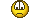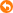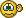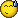# Java

 public class num1{         public static void main (String ars[]){                 int i;                 int  sum=0;                 i=1;                 while  (i<=10){                         sum +=i;                         i++;                 }                 System.out.println("Sum from1 to 10 is "+sum);         } } I don't know the meaning of the code.....I ask for the help原帖由 bus22004 於 2009-10-27 22:08 發表public class num1{         public static void main (String ars[]){                 int i;                 int  sum=0;                 i=1;                 while  (i Calculate the sum of 1,2,3,4,5,6,7,8,9,10 using while, they will increase 1 to variable "i" in each loop and add variable "i" to variable "sum", Sorry for my poor English and i hope i can help you some原帖由 ioptional 於 2009-10-27 22:21 發表Calculate the sum of 1,2,3,4,5,6,7,8,9,10 using while, they will increase 1 to variable "i" in each loop and add variable "i" to variable "sum", Sorry for my poor English and i hope i can help you so ... what is the mean of sum+=i?thankyou
 原帖由 bus22004 於 2009-10-27 22:32 發表what is the mean of sum+=i?thankyou it's the short hand for sum = sum + i you should study the operator chapter carefully